Automation, Control and Intelligent Systems
Volume 2, Issue 6-1, December 2014, Pages: 1-9

Speed control of a DC motor using Controllers

Md Akram Ahmad1, Kamal Kishor2, Pankaj Rai3

1Electronics and Communication Engineering Department, Maryland Institute of Technology and Management, Jamshedpur, India

2Electronics and Communication Engineering Department, Ramgovind Institute of Technology, Koderma, India

3Electrical Engineering Department, BIT Sindri, Dhanbad, India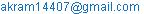(Md. Akram  Ahmad)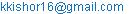(Kamal Kishor)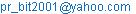(Dr. Pankaj Rai)

Md Akram Ahmad, Kamal Kishor, Pankaj Rai. Speed Control of a DC Motor Using Controllers. Automation, Control and Intelligent Systems. Special Issue: Impact of Gesture Recognition in the Technological Era. Vol. 2, No. 6-1, 2014, pp. 1-9. doi: 10.11648j.acis.s.2014020601.11

Abstract: This paper describes the speed control of a DC shunt motor using conventional controllers (PID, IMC) and Fuzzy Logic controller based on Matlab Simulation program. A mathematical model of the process has been developed using real plant data and then conventional controllers and Fuzzy logic controller has been designed. A comparative analysis of performance evaluation of all controllers has been done.

Keywords: PID Controller, IMC, FLC, DC Motor

Contents

1. Introduction

DC motors are widely used in industrial applications, robot manipulators and home appliances, because of their high reliability, flexibility and low cost, where speed and position control of motor are required. This paper deals with the performance evaluation of different types of conventional controllers and intelligent controller implemented with a clear objective to control the speed of separately excited DC motor.

PID controllers are commonly used for motor control applications because of their simple structures and intuitionally comprehensible control algorithms. Controller parameters are generally tuned using Ziegler-Nichols frequency response method . Ziegler-Nichols frequency response method is usually used to adjust the parameters of the PID controllers. However, it is needed to get the system into the oscillation mode to realize the tuning procedure. But it’s not always possible to get most of the technological plants into oscillation .

In process control, model based control systems are mainly used to get the desired set points and reject small external disturbances. The internal model control (IMC) design is based on the fact that control system contains some representation of the process to be controlled then a perfect control can be achieved. So, if the control architecture has been developed based on the exact model of the process then perfect control is mathematically possible .

Fuzzy logic control (FLC) is one of the most successful applications of fuzzy set theory, introduced by L.A Zadeh in 1973 and applied (Mamdani 1974) in an attempt to control system that are structurally difficult to model. Since then, FLC has been an extremely active and fruitful research area with many industrial applications reported . In the last three decades, FLC has evolved as an alternative or complementary to the conventional control strategies in various engineering areas.

Analysis and control of complex, nonlinear and/or time-varying systems is a challenging task using conventional methods because of uncertainties. Fuzzy set theory  which led to a new control method called Fuzzy Control which is able to cope with system uncertainties. One of the most important advantages of fuzzy control is that it can be successfully applied to control nonlinear complex systems using an operator experiences or control engineering knowledge without any mathematical model of the plant .

2. DC Motor

Direct current (DC) motors convert electrical energy into mechanical energy through the interaction of two magnetic fields. One field is produced by a magnet of poles assembly, the other field is produced by an electrical current flowing in the motor windings. These two fields result in a torque which tends to rotate the rotor.Figure 1.  DC shunt motor model.

The armature voltage equation is given by: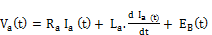(1)

Equation for back emf of motor will be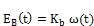(2)

Now the torque balance equation will be given by: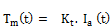(3)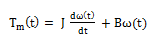(4)

Where,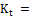Torque constant (Nm/A)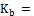back emf constant (Vs/rad)

Let us combine the upper equations together: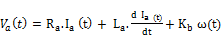(5)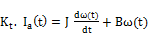(6)

Taking Laplace Transform of (5) & (6), we get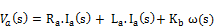(7)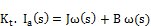(8)

If current is obtained from (8) and substituted in (7) we have…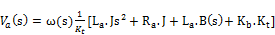(9)

Then the relation between rotor shaft speed and applied armature voltage is represented by transfer function: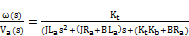(10)

This is the transfer function of the DC motor.

Consider the following values for the physical parameters [7,8]

Armature inductance (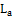) = 0.5 H

Armature resistance (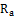) = 1Ω

Armature voltage (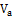) = 200 V

Mechanical inertia (J) = 0.01 Kg.m2

Friction coefficient (B) = 0.1 N-m/rad/sec

Back emf constant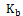= 0.01 V/rad/sec

Motor torque constant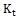= 0.01N.m/A

Rated speed = 1450 rpm

Based on the data book, the transfer function is as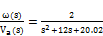(11)

3. Proportional-Integral-Derivative (PID) Controller

PID controllers are probably the most widely used industrial controller. In PID controller Proportional (P) control is not able to remove steady state error or offset error in step response. This offset can be eliminated by Integral (I) control action. Integral control removes offset, but may lead to oscillatory response of slowly decreasing amplitude or even increasing amplitude, both of which are error, initiates an early correction action and tends to increase stability of system.

Ideal PID controller in continuous time is given as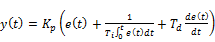(12)

Laplace domain representation of ideal PID controller is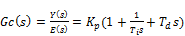(13)

Ziegler and Nichols proposed rules for determining values of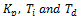based on the transient response characteristics of a given plant. Closed loop oscillation based PID tuning method is a popular method of tuning PID controller. In this kind of tuning method, a critical gain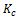is induced in the forward path of the control system. The high value of the gain takes the system to the verge of instability. It creates oscillation and from the oscillations, the value of frequency and time are calculated. Table 1 gives experimental tuning rules based on closed loop oscillation method [2,9].

Table 1. Closed loop oscillation based tuning methods.

 Type of Controller P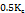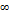PI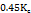PID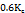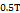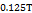From the Closed loop oscillation method,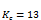and T = 2 sec, which implies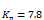,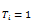and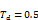Usually, initial design values of PID controller obtained by all means needs to be adjusted repeatedly through computer simulations until the closed loop system performs or compromises as desired. These adjustments are done in MATLAB simulation.

4. Internal Model Control (IMC)

The theory of IMC states that "control can be achieved only if the control system encapsulates, either implicitly or explicitly, some representation of the process to be controlled".

The Internal Model Controller is based on the inverse of the process model we are trying to control. If we cascade the process transfer function with a controller which is the exact inverse of the process, then effectively the gain becomes unity and we have perfect set-point tracking . The main feature of internal model controller is that the process model is in parallel with the actual process.

Figure (2) shows the scheme of IMC. Internal model controller provides a transparent framework for control system design and tuning.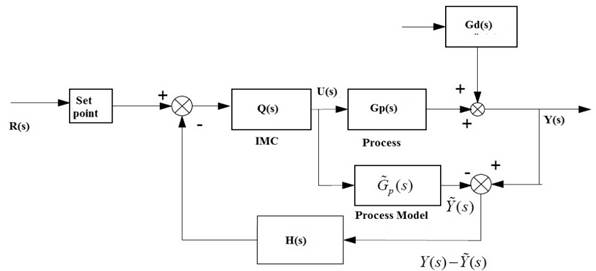Fig 2. Internal module control scheme.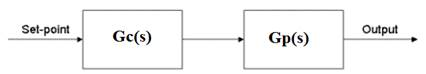Fig 3. Equivalent Block diagram of IMC

A controller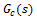has been used to control the process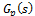. Suppose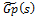is a model of.

Where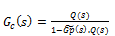(14)

And if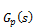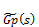(15)

the model is an exact representation of process, then it is clear that the output will always equal to set point. Notice that this ideal control performance is achieved without feedback.

Designed the IMC controller as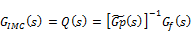(16)

Where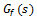is a low pass function defined as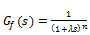(17)

Here we consider 2nd order low pass filter (n = 2).

Thus,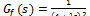,

Where λ is closed loop time constant.

Now,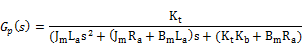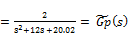(18)

A good rule of thumb is to choose λ to be twice fast as open loop response.

λ=0.9 implies,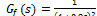Thus,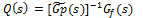becomes,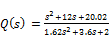(19)

Hence, from equation (14)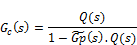Implies,(20)

5. Fuzzy Logic Controller (FLC)

Table 2. Linguistic variables.

 NB NM NS Z PB PM PS Big negative Medium negative Small negative Zero Big positive Medium positive Small positive

Table 3. Rule base for fuzzy logic controller.

 ce e NB NM NS Z PS PM PB NB NB NB NB NB NM NS Z NM NB NB NB NM NS Z PS NS NB NB NM NS Z PS PM Z NB NM NS Z PS PM PB PS NM NS Z PS PM PB PB PM NS Z PS PM PB PB PB PB Z PS PM PB PB PB PB

The fuzzy controllers are designed with two input variables, error and change of error and one output variable. The Mamdani based fuzzy inference system uses linear membership function for both inputs and outputs . For the fuzzy logic controller the input variables are error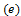and rate (change) of error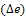, and the output variable is controller output. Triangular membership functions are used for input variables and the output variable. Each variable has 7 membership functions. Thus, there were total 49 rules generated. The universe of discourse of error, rate of error and output are [120,120], [120,120] and [180,180] respectively. The rule base framed for DC motor is tabulated in Table 3.

The structure of the rule base provides negative feedback control in order to maintain stability under any condition. Linguistic variables for error, rate of error and controller output are tabulated in table 2.

Fig 4, 5 and 6 shows membership functions of different variables implemented in FIS editor   in MATLAB toolbox.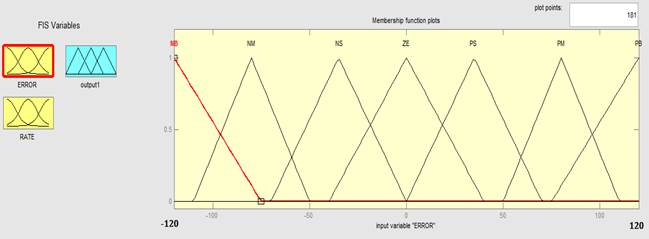Fig 4. Membership functions for input-1(error).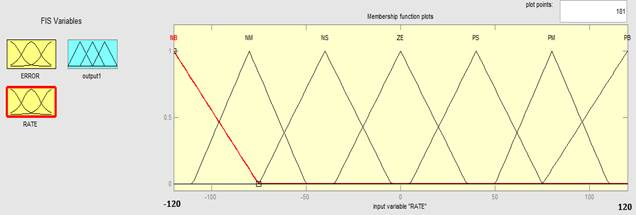Fig 5. Membership functions for input-2(change of error).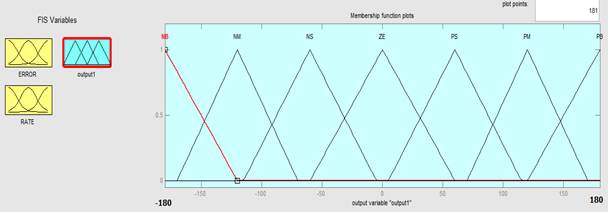Fig 6. Membership functions for output.

6. Simulation

The simulations for different control mechanism discussed above were carried out in Simulink in MATLAB and simulation results have been obtained.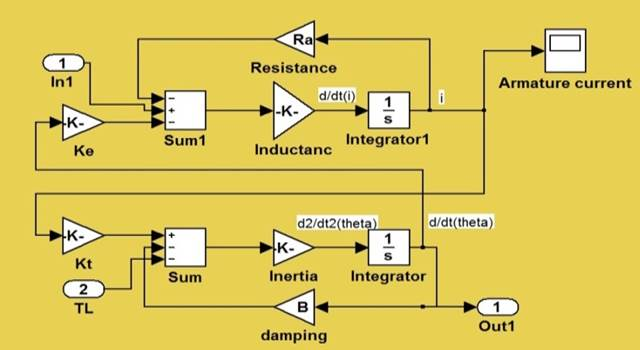Fig 7. Simulink model of DC motor.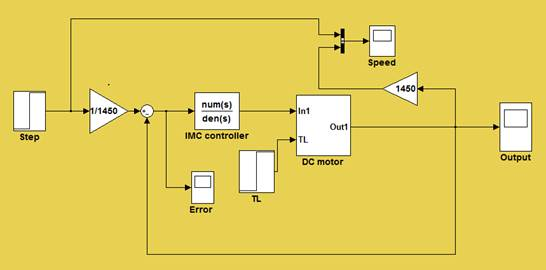Fig 9. IMC Controller Simulink model.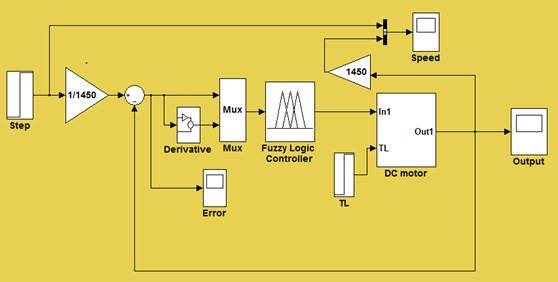Fig 10. Simulink model of FLC Controller.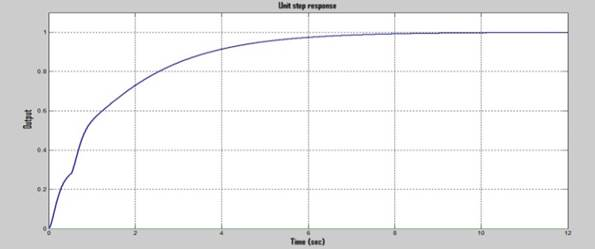Fig 11. Unit step response of PID Controller.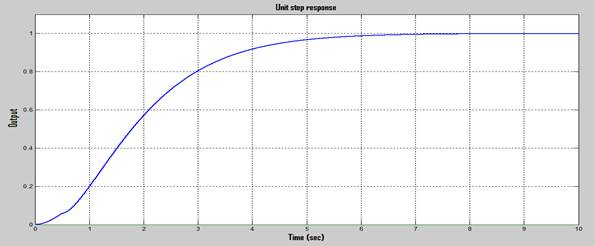Fig 12. Unit step response of IMC.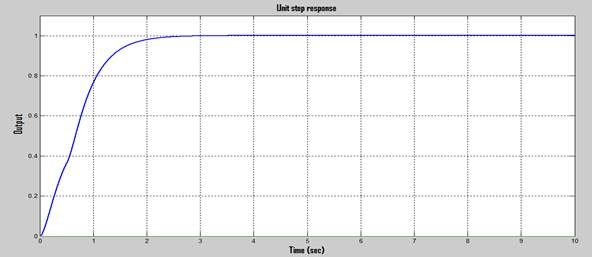Fig 13. Unit step response of FLC.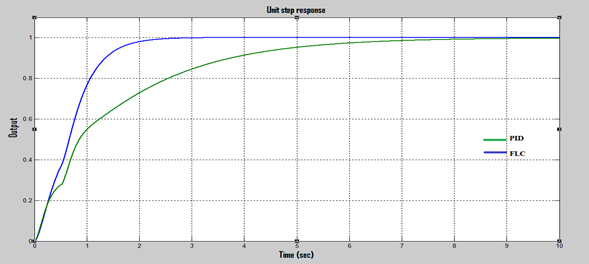Figure 14. Comparison of unit step response of PID and FLC controllers.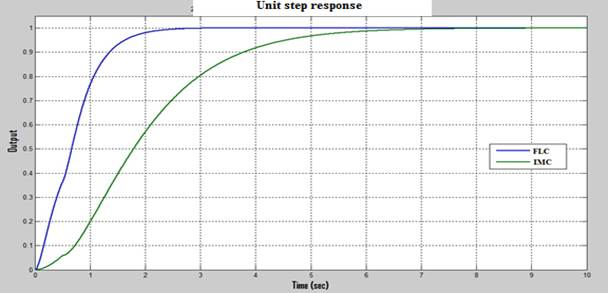Fig 15. Comparison of unit step response of IMC and FLC controllers.

7. Result and Discussion

To evaluate the performance of the different controllers, the maximum overshoot, the settling time and the rise time of step response has been analyzed.

Table 4 shows the comparison of parameters calculated from unit step response

Table 4. Comparison of different parameters in controllers

 Controllers Maximum Overshoot (%) Settling time (sec) Rise time (sec) PID 2.1 7.2 3.65 IMC 0 6.9 2.5 FLC 0 2.3 1.8

The feedback controller (PID controller) gives 2.1% peak overshoot with settling time of7.2 sec. The peak overshoot is in a higher side. To compensate the high peak overshoot, model based controller (internal model controller) was designed. The internal model controller (IMC) reduces the peak overshoot to 0% and reduces the settling time to 6.9 sec. To further improve the response, Fuzzy logic controller having seven membership functions has been designed. The designed fuzzy logic controller gives a peak overshoot of 0% (no overshoot) and reduces the settling time to 2.3 sec.

8. Conclusion

In this paper, comparative studies of performance of different conventional controllers and fuzzy logic controller has been studied. According to the comparison of results of the simulations, it is found that the Fuzzy Logic Controller is better than conventional controllers namely PID and IMC.

Hence it is concluded that the proposed Fuzzy Logic Controller provides better performance characteristics and improve the control of DC motor.

References

1. Husain Ahmed and Gagansingh, "Controlling of D.C. Motor using Fuzzy Logic Controller", Conference on Advances in Communication and Control Systems 2013 (CAC2S 2013)
2. Katsuhiko Ogata, "Modern Control Engineering".   5thedition  2010.
3. M.Saranya and D.Pamela, "A Real Time IMC Tuned PID Controller for DC Motor", International Journal of Recent Technology and Engineering, ISSN: 2277-3878, Volume-1, Issue-1, April 2012
4. H.X.Li and S.K.Tso, "Quantitative design and analysis of Fuzzy Proportional- Integral- Derivative Control- a Step TowardsAutotuning", International journal of system science, Vol.31, No.5, 2000, pp.545-553.
5. Zadeh, L. A., Fuzzy Sets. Information and Control, 8, 338-353, 1965.
6. Assilian, S. and Mamdani, E.H., An Experiment in Linguistic Synthesis with a Fuzzy Logic Controller. International Journal of Man-Machine Studies, 7(1), 1-13, 1974.
7. K.Venkateswarlu and Ch. Chengaiah "Comparative study on DC motor speed control using various controllers" Volume 1 , Issue 6 / Dec 2013
8. AlokRanjan Singh and V.K. Giri "Design and Analysis of DC Motor Speed Control by GA Based Tuning of Fuzzy Logic Controller" International Journal of Engineering Research & Technology (IJERT), Vol. 1 Issue 5, July – 2012
9. KiamHeongAng, Gregory Chong and Yun Li, "PID Control System Analysis, Design, and Technology," IEEE Trans., Control Syst. Technol., vol. 13, no. 4, pp. 559-576, Ju12005.
10. Zhicheng Zhao, Jianggang Zhang and MingdongHou, "An adaptive IMC-PID control scheme based on neural network," presented at the IEEE conference, 2009.
11. AlokRanjan Singh and V.K. Giri "Compare and simulation of speed control of DC motor using PID and Fuzzy controller"VSRD International Journal of Electrical, Electronics & Communication Engineering, Vol. 2 No. 10 October 2012
12. Larsen P.M., Industrial application of Fuzzy Logic Control,academic press, inc., may, 1979.
13. Zadeh L.A., Fuzzy relation Equations and Applications to Knowledge Engineering, Kluwer AcademicPublishers, Holland, 1989

 Contents 1. 2. 3. 4. 5. 6. 7. 8.
Article ToolsAbstractPDF(2990K)